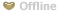Eng | Рус | Fra | Esp | Deu | 中文

# Dating with Kate (poustovit), girl from kiev, Ukraine• First Name: Kate
• 54 y/o female, Zodiac: Pisces
• kiev, Ukraine
• English(Intermediate), Russian(Fluent)
• 1 child
• Last online: 2017-12-24 18:48:14
• Nickname: poustovit
• ID: 1000240952
 Private details and contact information Personal details Sex female Children 1 child Want children I will tell you later Height 5'4" - 5'5" (161-165cm) Body type Attractive Ethnicity Mixed Religion Christian Marital status Single Education High School graduate Income Smoker Rarely Drinker Rarely Details of the person you are looking for I look for a male Looking for an age range 43-52 Looking for a height Looking for a body type Relationship Activity Partner, Friendship, Marriage
 Description: Let there will be the peace and love. Love each other and be happy)))))))))))))))))))))))))))))) ))))))))))))))))))))))))))))))))))) ) ))))))))))))))))))))))))))))))))))) ))))))))))))))))))))))))))))))))))) ) ))))))))))))))))))))))))))))))))))) ) ))))))))))))))))))))))))))))))))))) ) ))))))))))))))))))))))))))))))))))) ) ))))))))))))))))))))))))))))))))))) ) ))))))))))))))))))))))))))))))
 Ideal match description:
TOP profiles of most popular Ukrainian girls: